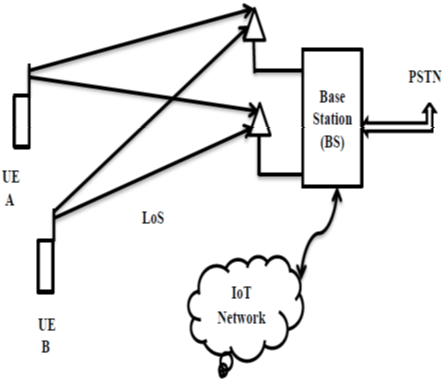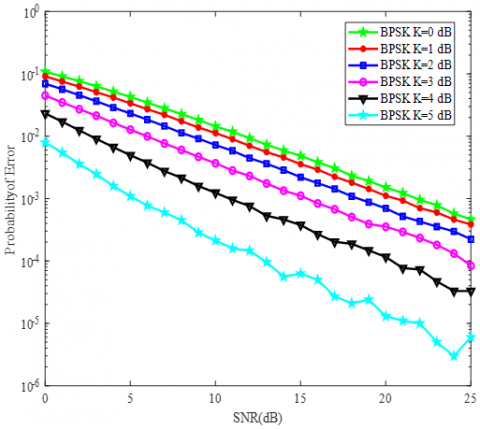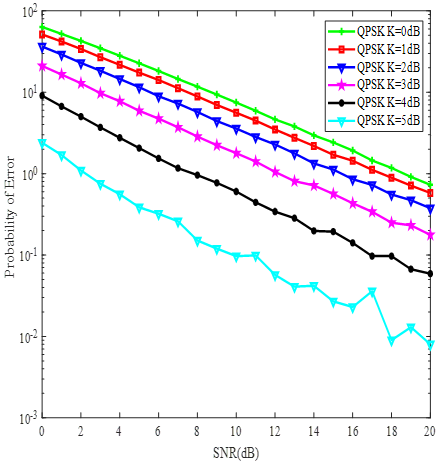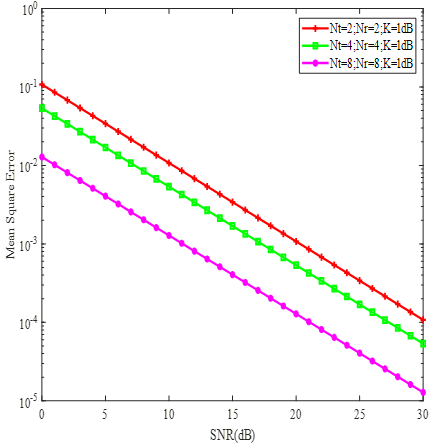# Physical (PHY) Layer Analysis of Data Transmission in MIMO Wireless Networks in Line- of-Sight (LoS) Environments

Physical (PHY) Layer Analysis of Data Transmission in MIMO Wireless Networks in Line- of-Sight (LoS) Environments

M. PremkumarM. Arun S. Sathiya Priya R. Prathipa D. Gurupandi

Department of ECE, Panimalar Institute of Technology, Chennai 600123, Tamil Nadu, India

Corresponding Author Email:
ermpremkumar@yahoo.co.in
Page:
210-214
|
DOI:
https://doi.org/10.18280/mmep.090126
3 December 2021
|
Accepted:
27 January 2022
|
Published:
28 February 2022
| Citation

OPEN ACCESS

Abstract:

This research paper presents the Physical (PHY) layer analysis of multiple input multiple output (MIMO) systems in line-of-sight environments between a user equipment (UE) and a base station when an information data is transmitted. The data transmission in wireless networks often takes place in non-line-of-sight in urban environments and Line-of-Sight (LoS) in rural environments depending on the bandwidth, power constraints. Using MIMO technology, the data transmission takes place with increased number of transmit and receive antennas which results in reduced signal to noise ratio (SNR) requirement. In this research work, simulation for PHY layer metric of probability of error, mean square error (MSE) for data transmission in MIMO wireless networks is obtained Matrix Laboratory (MATLAB) for LoS environment which follows Rician distribution. Probability of error reduces to a significant extent when the Rician factor increases as the line-of-sight component prevails which also takes lesser power in the lower SNR limits. Further, in MIMO systems also the probability of error and MSE reduces in concatenation with single antenna terminals thereby exploring power control a significant possibility for implementation in massive MIMO and millimeter wave communication systems for 5G and 6G systems.

Keywords:

physical layer, multiple input multiple output, line-of-sight, probability of error, mean square error

1. Introduction

Wireless networks in Physical layer perspective  are getting increasingly significant due to advancing data rates of the order of Gbps due to multiple input multiple output (MIMO)in the forthcoming deployment of 5G and 6G systems [2, 3] irrespective of data transmission as audio, image and video signals. MIMO wireless networks are advantageous in the aspects of diversity gain, spectral efficiency, reliability and coverage. However, when MIMO technology is extended to an array of antennas in the base station (BS) as that of massive MIMO, millimeter wave (mmWave), technologies  it can reap huge benefits in PHY layer attributes of lower probability of error, reduced signal to noise ratio and ultimately leading to enhanced data rates. Propagation channel statistics for MIMO wireless networks undergo multipath fading  either in frequency non selective or frequency selective fading channel which play a role in data transmission. Irrespective of channel statistics always a Line-of-Sight (LoS) [6, 7] path is needed for efficient information transfer between a transmitter node and receiver node in a wireless transceiver chain such as MIMO wireless networks. Though predominantly NonLine-of-Sight (NLoS) path exists in practical situations, LoS channel also gains importance in applications in microwave frequency ranges GHz, WiFi networks, millimeter wave propagation  where the channel is considered to undergo Rician fading given by line of sight component KdB[9, 10].Further in Internet of Things (IoT) based smart cities which uses sensors in microwave ranges  and other electronic circuitry of combinational circuits  and sequential circuits there lies a possibility of having LoS in a MIMO wireless network which advocates the need for analysis of MIMO wireless networks.

Research literatures for MIMO wireless networks in LoS channels specified by Rician fading can be obtained from the works [14-19] and PHY layer analysis gains utmost importance. In the research  MIMO channel model which are having K adjustable factors are analyzed. A Rician channel with multiple antennas in a jamming aware decision fusion system is addressed in the contribution work . The present-day technologies of millimeter wave propagation in line-of-sight MIMO channels with measured results are dealt in the research . Also, Harris et al.  presents the number of rates which can be achieved and required overhead during training for massive MIMO channel where massive MIMO infers to usage of antenna arrays at the base station. Moreover, considering security aspects at the Physical layer for Rician fading, chaotic based data transmission for non-orthogonal multiple access (NOMA) networks are presented . Wireless data traffic in millimeter wave massive MIMO communication is dealt in , where energy efficiency of small number of cells at higher frequency ranges in GHz is presented. Though all the above research works stated are excellent in terms of research results obtained, this research work presents MIMO wireless networks in LoS channels which undergoes Rician distribution which will be useful for researchers working in the wireless domain sector. Simulation Results are obtained with multiple antennas at the transmitter and receiver with different Rician factor KdBwhich always seems to be a dominant component which contributes to reduced probability of error, lower signal to noise ratio and thereby aid less power control at the transmitting node. Mean Square Error (MSE) analysis for MIMO wireless networks is also obtained in LoS environment. Figure 1 shows the considered MIMO wireless network with base station, IoT network and Public Switched Telephone Network (PSTN) arrangement.

This research article is written in the following sections. Section 1 gives an insight into MIMO wireless network with LoS environment. Section 2 mathematically portrays system model for data transmission in single antenna configuration. Section 3 gives system model for MIMO data transmission with imperfect channel estimates. Section 4 presents the simulation results for PHY layer metric probability of error using BPSK, QPSK with singe antenna and MIMO in Rician environment for BPSK scheme and mean square error (MSE) for imperfect channel conditions with MIMO configuration. Section 5 gives the conclusions for the research paper.

## 1.pngFigure 1. MIMO wireless network in LoS environment

2. System Model for Data Transmission

Consider a wireless network having a user equipment (UE) A and user equipment B which transmits a data signal d to the base station (BS)following binary phase shift keying (BPSK) in a Line-of-Sight (LoS) environment. Mathematically the received signal z is expressed as:

$z=l d+w$       (1)

where, d is the data signal, w is additive white Gaussian noise (AWGN) with mean zero $\mu_{x}$ and variance $\sigma_{x}^{2}$, l is the line-of-sight channel given $l=\beta_{0} e^{j \emptyset_{0}}+K_{d B}$, where $\beta_{0}$ is the attenuation constant which is Rayleigh distributed, $\emptyset_{0}$ is the phase constant which is uniformly distributed in the interval (0,2π). Further, Rician distribution is given by the following probability density function (PDF).

$f_{l}(l)=\left\{\begin{array}{cc}\frac{l}{\sigma^{2}} e^{\frac{-\left(l^{2}+A^{2}\right)}{2 \sigma^{2}}} I_{0}\left(\frac{A l}{\sigma^{2}}\right) ; & \text { for }(A \geq 0 ; l \geq 0) \\ 0 ; & \text { for }(l<0)\end{array}\right\}$       (2)

Now extending (1) to MIMO wireless network scenario, with multiple antennas at the transmitter Ntand multiple antennas at the receiver Nr . The received signal at a particular time instant t1 uses encoding method as space time codes  and it is formulated as:

$z_{1}=l_{1} d_{1}+l_{2} d_{2}+w_{1}$       (3)

Similarly, the received signal  at time instant t2, t3, t4 as per space time coding it is written as:

$Z_{2}=-l_{1} d_{1}^{*}+l_{2} d_{1}^{*}+w_{2}$       (4)

$Z_{3}=l_{3} d_{1}+l_{4} d_{2}+w_{3}$      (5)

$z_{4}=-l_{3} d_{1}^{*}+l_{4} d_{2}+w_{4}$      (6)

The signals between the different antennas do not affect each other as they are independent and it is uncorrelated. Using maximal ratio combining (MRC) scheme and maximum likelihood decoding technique the estimate  of the received signals for the two received signals are given as:

$\tilde{d}_{1}=l_{1}^{*} z_{1}+l_{2} z_{1}^{*}+l_{3}^{*} z_{2}+l_{3} z_{3}^{*}$      (7)

$\tilde{d}_{2}=l_{2}^{*} z_{1}-l_{1} z_{1}^{*}+l_{4}^{*} z_{2}-l_{3} z_{3}^{*}$      (8)

Moreover, for BPSK using the Euclidean distance rule  it is,

$d^{2}\left(\tilde{d}_{0}, d_{i}\right) \leq d^{2}\left(\tilde{d}_{0}, d_{k}\right) ;$ for all $i \neq k$       (9)

Based on the above expressions of (8) and (9) the probability of error for LoS MIMO wireless network can be simulated for the modulation scheme of BPSK. The error probability can also be obtained under different modulation schemes depending upon the number of bits which it takes as that of 2 bits for quadrature phase shift keying (QPSK) and also based on number of subcarriers for orthogonal frequency division multiplexing (OFDM) based modulation schemes.

3. System Model for Data Transmission with Imperfect Channel Estimates

Based on the analysis of (1) imperfect estimates for MIMO wireless networks can be obtained by rewriting the expression as:

$\boldsymbol{Z}=\boldsymbol{L D}+\boldsymbol{W}$      (10)

Since the channel state information (CSI) is always not available it needs to be estimated from the MIMO received signal model as in (10). CSI can be obtained by estimation algorithms like least squares (LS) algorithm , minimum mean square error (MMSE) algorithm and maximum likelihood (ML)approaches. To obtain a MIMO channel estimate we follow by considering an objective function F which needs to be optimized for L the Nr x Nt MIMO channel matrix. To derive the MIMO channel estimate of L^ consider the objective function F.

$F=(\boldsymbol{Z}-\boldsymbol{L} \boldsymbol{D})^{2}$      (11)

On applying partial differentiation relating to the channel matrix L it is:

$\frac{\partial F}{\partial L}=2(\boldsymbol{Z}-\boldsymbol{L} \boldsymbol{D}) \boldsymbol{D}$       (12)

The objective function has square of white Gaussian noise. The basis is that white Gaussian noise has zero mean and hence it is considered to be negligible which is zero and not considered for further analysis in terms of expressions. To get the minimum value it is equated to zero and it is given as:

$\frac{\partial F}{\partial L}=2(\boldsymbol{Z}-\boldsymbol{L D}) \boldsymbol{D}=0$       (13)

On further simplification with rearrangement, it is:

$\boldsymbol{Z}=0+\hat{L}_{l s} \boldsymbol{D}$       (14)

The least square estimate of the MIMO channel matrix is:

$\widehat{L}_{l s}=Z D^{-1}$       (15)

The above expression (15) represents MIMO channel matrix obtained using least squares estimation procedure for finding out the channel state information in imperfect situation which can be helpful for beamforming, error estimate analysis with mean square error (MSE) metric. Further MMSE and ML approaches can also be considered as an extension work for finding MIMO channel matrix. Also, the MIMO channel matrix under Rician fading conditions which exhibit LoS can also be correlated or as that of uncorrelated situations based on the correlation coefficient. The mean square error  for least squares in MIMO can be obtained as follows considering (16).

$M S E_{\text {mimo }}=\operatorname{tr}\left(E\left(\left(\boldsymbol{L}-\widehat{\boldsymbol{L}}_{l s}\right)\left(\boldsymbol{L}-\widehat{\boldsymbol{L}}_{l s}\right)^{H}\right)\right)$       (16)

Using the equation of (15) and (10) in (16) it reaches to,

$\hat{L}_{l s}=(Z D)^{-1}(L D+W)$       (17)

Now on expanding (17) it is written in the following manner:

$\widehat{\boldsymbol{L}}_{l s}=(\boldsymbol{Z} \boldsymbol{D})^{-\mathbf{1}}(\boldsymbol{L} \boldsymbol{D})+\left((\boldsymbol{Z} \boldsymbol{D})^{-\mathbf{1}} \boldsymbol{W}\right)$       (18)

In the above expression of (18) the term with W constitutes additive white Gaussian noise (AWGN) with mean zero and variance unity. The other terms with channel LoS are not related to the error and hence it is Error $_{L S}=\left(L-\hat{L}_{l s}\right)$ which is further expressed as:

$Error _{L S}=(Z D)^{-1} W$       (19)

The mean square error using (19) can be written as:

$M S E_{\text {mimo }}=\operatorname{tr}\left(E\left(\right.\right.$ Error $_{L S}$ Error $\left.\left.^{H}\right)\right)$       (20)

Finally, the MSE expression in MIMO environment is found to be as in (21):

$M S E_{\text {mimo }}=\operatorname{tr}\left(E\left((Z D)^{-1} W\left((Z D)^{-1} W\right)^{H}\right)\right)$      (21)

4. Simulation Results & Discussions

This section analyses the obtained PHY layer simulation results for probability of error for MIMO wireless networks and mean square error (MSE). Probability of error against signal to noise ratio (SNR) is simulated in MATLAB under Line-of-Sight (LoS) path which is Rician distributed. Rician distribution is given by the various KdB values which represent Line-of-Sight components or Rician factor dominant components for KdB values of 0 dB, 1 dB, 2 dB, 3 dB, 4 dB and 5 dB. For single antenna wireless network and MIMO wireless network with modulation schemes are analyzed under Monte Carlo runs with 1000 iterations.

Figure 2 shows probability of error performance of line-of-sight environment with Rician distribution K factors for a probability of error of 10-3 under single antenna consideration with BPSK modulation scheme. As observed from the simulation results when the line-of-sight components KdBincreases from 0 dB, 1 dB, 2 dB, 3 dB, 4 dB and 5 dB the signal to noise ratio gradually decreases. This reduction in SNR is due to impact of the LoS component which can be useful for design of wireless networks with single antenna terminals in user equipment relating to power control. Similarly, Figure 3 shows the probability of error performance using QPSK modulation scheme and same analysis infers that LoS component contributes to reduced signal to noise ratio (SNR). Table 1 shows the obtained results Single Antenna BPSK wireless networks.

## 2.pngFigure 2. Signal to noise ratio vs probability of error for line-of-sight environments for different line-of-sight (KdB) Factors using BPSK modulation scheme

## 3.pngFigure 3. Signal to noise ratio vs probability of error for line-of-sight environments for different line-of-sight (KdB) factors for QPSK modulation scheme

Table 1. Probability of error single antenna BPSK wireless networks

 Single antenna wireless networks LoS environment Rician factor KdB Probability of error SNR(dB) 0 dB 10-3 22 dB 1 dB 10-3 20 dB 2 dB 10-3 18dB 3 dB 10-3 15dB 4 dB 10-3 12 dB 5 dB 10-3 6dB

Figure 4 shows MIMO wireless network probability of error performance against SNR with various line of sight factors KdB. The SNR requirement is very less and probability of error reduces to a greater extent when the LoS component progresses from 0 dB, 1 dB, 2 dB, 3 dB, 4 dB where probability of error falls below 10-4, which shows the importance of having multiple antennas at the transmitter and receiver which can be a user equipment and receiver can be a base station. Table 2 gives Probability of error analysis of MIMO wireless networks.

## 4.pngFigure 4. Signal to noise ratio vs probability of error for line-of-sight environments for different line-of-sight (KdB) factors in MIMO Environment

Table 2. Probability of error for MIMO wireless networks in LoS environment

 MIMO wireless networks LoS environment Rician factor KdB Probability of error SNR (dB) 0 dB 10-4 4 dB 1 dB 10-4 3 dB 2 dB 10-4 2 dB 3 dB 10-4 <1 dB 4 dB 10-4 < 1dB

Figure 5 shows mean square error when the number of antennas Nt and Nr are considered to be 2,4 and 8. To achieve a MSE of 10-2, 2 transmit and receive antennas take 11 dB SNR, 4 antenna configurations take 9 dB SNR and 8 antenna configurations take less than 1 dB SNR. The reduction in SNR is due to the diversity gain due to MIMO configuration and when it comes to design of massive MIMO systems when employed in higher frequency ranges of the order of GHz, where power and bandwidth constraints it becomes highly significant.

## 5.pngFigure 5. Mean square error vs signal to noise ratio for line-of-sight environments for KdB in MIMO environment

5. Conclusion

The outcomes of the research paper it provides probability of error analysis, mean square error of MIMO wireless networks in LoS environment which undergo Rician distribution. The LoS channel contributes to reduced probability of error in the lower and higher SNR ranges which indicates the need for transmit power control in the user equipment side for data transmission towards base station irrespective of the modulation schemes employed. MSE analysis also suggests that on increasing the number transmit antennas and receiving the error reduces with significant reduction in SNR. The obtained results can be useful for analyzing 5G and 6G systems where data rates of the order of Gbps are used for data transmission and reception in physical layer analysis. In future this work can be extended to work on multiuser MIMO systems where interference analysis in the form of signal to interference noise ratio is an important performance metric.

References

 Liang, T., Li, W.L. (2009). The physical layer design for MIMO wireless communications system. In 2009 5th International Conference on Wireless Communications, Networking and Mobile Computing, Beijing, China, pp. 1-4. https://doi.org/10.1109/WICOM.2009.5300850

 Gangfada, I.A., Abdullahi, U.S., Ugweje, O., Koyunlu, G., Suleiman, H.U., Chijioke, C.E. (2020). An evaluation of the physical layer characterization of 5G networks. In 2020 4th International Conference on Trends in Electronics and Informatics (ICOEI), Tirunelveli, India, pp. 496-504. https://doi.org/10.1109/ICOEI48184.2020.9142986

 Saad, W., Bennis, M., Chen, M. (2019). A vision of 6G wireless systems: Applications, trends, technologies, and open research problems. IEEE Network, 34(3): 134-142. https://doi.org/10.1109/MNET.001.1900287

 Tariq, M.H., Chondroulis, I., Skartsilas, P., Babu, N., Papadias, C.B. (2020). mmWave massive MIMO channel measurements for fixed wireless and smart city applications. In 2020 IEEE 31st Annual International Symposium on Personal, Indoor and Mobile Radio Communications, London, UK, pp. 1-6. https://doi.org/10.1109/PIMRC48278.2020.9217375

 Hussain, G.A., Audah, L. (2020). BCH codes for 5G wireless communication systems over multipath fading channel. Indonesian Journal of Electrical Engineering and Computer Science, 17(1): 310-316. https://doi.org/10.11591/ijeecs.v17.i1.pp310-316

 Ferrand, P., Yang, S. (2016). Blind precoding in line-of-sight MIMO channels. In 2016 IEEE 17th international workshop on signal processing advances in wireless communications (SPAWC), Edinburgh, UK, pp. 1-5. https://doi.org/10.1109/SPAWC.2016.7536764

 Zhou, L., Ohashi, Y. (2015). Performance analysis of mmWave LOS-MIMO systems with uniform circular arrays. In 2015 IEEE 81st Vehicular Technology Conference (VTC Spring), Glasgow, UK, pp. 1-5. https://doi.org/10.1109/VTCSpring.2015.7146001

 Xue, Y., Zheng, X., Lau, V.K. (2020). Line-of-sight MIMO for high capacity millimeter wave backhaul in FDD systems. Journal of Communications and Information Networks, 5(2): 177-193. https://doi.org/10.23919/JCIN.2020.9130434

 Yang, H., Marzetta, T.L. (2017). Massive MIMO with max-min power control in line-of-sight propagation environment. IEEE Transactions on Communications, 65(11): 4685-4693. https://doi.org/10.1109/TCOMM.2017.2725262

 Kildal, P. S., Chen, X., Gustafsson, M., Shen, Z. (2014). MIMO characterization on system level of 5G microbase stations subject to randomness in LOS. IEEE Access, 2: 1062-1075. https://doi.org/10.1109 /ACCESS. 2014. 2358937

 Cetinkaya, O., Balsamo, D., Merrett, G.V. (2020). Internet of MIMO things: UAV-assisted wireless-powered networks for future smart cities. IEEE Internet of Things Magazine, 3(1): 8-13. https://doi.org/10.1109/IOTM.0001.1900064

 Jackson, B., Jayanthy, T. (2014). Determination of sucrose in raw sugarcane juice by microwave method. Indian Journal of Science and Technology, 7(5): 566-570.

 Sridevi, V., Jayanthy, T. (2014). Minimization of CNTFET ternary combinational circuits using negation of literals technique. Arabian Journal for Science and Engineering, 39(6): 4875-4890. https://doi.org/10.1007/s13369-014-1147-y

 Pratschner, S., Blazek, T., Zöchmann, E., Ademaj, F., Caban, S., Schwarz, S., Rupp, M. (2019). A spatially consistent MIMO channel model with adjustable k factor. IEEE Access, 7: 110174-110186. https://doi.org/10.1109/ACCESS.2019.2934635

 Ciuonzo, D., Aubry, A., Carotenuto, V. (2017). Rician MIMO channel-and jamming-aware decision fusion. IEEE Transactions on Signal Processing, 65(15): 3866-3880. https://doi.org/10.1109/TSP.2017.2686375

 Halsig, T., Cvetkovski, D., Grass, E., Lankl, B. (2017). Measurement results for millimeter wave pure LOS MIMO channels. In 2017 IEEE Wireless Communications and Networking Conference (WCNC), San Francisco, CA, USA, pp. 1-6. https://doi.org/10.1109/WCNC.2017.7925749

 Harris, P., Hasan, W.B., Liu, L., et al. (2018). Achievable rates and training overheads for a measured LOS massive MIMO channel. IEEE Wireless Communications Letters, 7(4): 594-597. https://doi.org/10.1109/LWC.2018.2799863

 Al-Musawi, I., Al-Hussaibi, W., Ali, F. (2021). Chaos-based physical layer security in Noma networks over Rician fading channels. In 2021 IEEE International Conference on Communications Workshops (ICC Workshops), Montreal, QC, Canada, pp. 1-6. https://doi.org/10.1109/ICCWorkshops50388.2021.9473862

 Al-Heety, A.T., Islam, M.T., Rashid, A.H., Ali, H.N.A., Fadil, A.M.,Arabian, F. (2020). Performance evaluation of wireless data traffic in mm wave massive MIMO communication. Indonesian Journal of Electrical Engineering and Computer Science, 20(3): 1342-1350. https://doi.org/10.11591/ijeecs.v20.i3.pp1342-1350

 Alamouti, S.M. (1998). A simple transmit diversity technique for wireless communications. IEEE Journal on Selected Areas in Communications, 16(8): 1451-1458. https://doi.org/10.1109/49.730453

 Steven, M.K. (2003). Fundamentals of Statistical Signal Processing, Volume I: Estimation Theory/Volume II: Detection Theory.